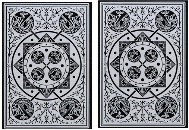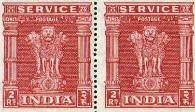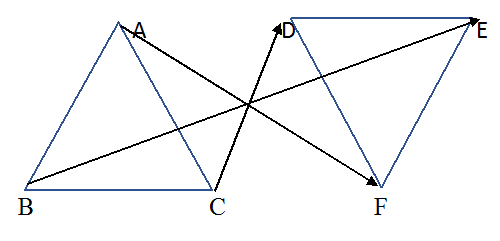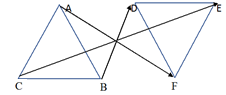# Excercise 7.1 Congruence-of-Triangles- NCERT Solutions Class 7

## Chapter 7 Ex.7.1 Question 1

Complete the following statements:

a) Two-line segments are congruent if ___________.

b) Among two congruent angles, one has a measure of $$70^\circ$$; the measure of the other angle is ___________.

c) When we write $$∠A = ∠B,$$ we actually mean ___________.

### Solution

Steps:

a) they have same length.

b) $$70^\circ$$

c) $$m ∠A = m ∠B$$ (m stands for measure of the angle)

## Chapter 7 Ex.7.1 Question 2

Give any two real-life examples for congruent shapes.

### Solution

Steps:

a) Cards of same playing card deck.b) Same postage stamps.## Chapter 7 Ex.7.1 Question 3

If $$ΔABC ≅ ΔFED$$ under the correspondence $$ABC ↔ FED,$$ write all the corresponding congruent parts of the triangles.

### Solution

What is the known?

Two triangles which are congruent.

What is the unknown?

Triangles $$ABC$$ and $$FED$$ are congruent $$(ΔABC ≅ ΔFED)$$ under the correspondence $$ABC ↔ FED$$

Reasoning:

Two congruent triangles have exactly the same three sides and exactly the same three angles. According to the given correspondence, triangle$$ABC$$ can be placed on triangle$$FED$$ in such a way that $$A$$ falls on $$F$$, $$B$$ falls on $$E$$ and $$C$$ falls on $$D$$. So, while taking about congruence of triangles, measure of sides, measure of angles and matching of vertices are equally important.

Steps:

Given, $$ΔABC ≅ ΔFED$$ under the correspondence $$ABC ↔ FED.$$ The correspondence is shown with arrow in the following figure.It is clear from the figure that

\begin{align}& \angle A\leftrightarrow \! \angle F,\!\angle \! B\leftrightarrow \! \angle E, \!\angle C \! \leftrightarrow \! \angle D \\& \overline{AB}\ \! \leftrightarrow \! \overline{FE}, \!\overline{BC}\ \! \leftrightarrow \! \overline{ED}, \!\overline{AC}\ \! \leftrightarrow \! \overline{FD} \\ \end{align}

## Chapter 7 Ex.7.1 Question 4

If $$ΔDEF ≅ ΔBCA$$, write the part(s) of $$ΔBCA$$ that correspond to

$$\left( \text{i} \right)\,\,\angle E\\\left( \text{ii} \right)\text{ }\overline{EF}~\\\left( \text{iii} \right)\,\,\angle F~\\\left( \text{iv} \right)\overline{\text{ }DF}.$$

### Solution

What is the known?

Two $$ΔDEF$$ and $$ΔBCA$$ which are congruent.

What is the unknown?

The part(s) of $$ΔBCA$$ that correspond to different angles and sides.

Reasoning:

This question is based on the concept of congruence of triangles. As it is given in the question two triangles $$DEF$$ and $$BCA$$ are congruent. We can place triangle $$DEF$$ on $$BCA$$ such that $$D$$ falls on $$B$$, $$F$$ falls on $$A$$, $$F$$ falls on $$A$$ and $$D$$ falls on $$C$$.

Steps:

Since, $$ΔDEF ≅ ΔBCA$$

For better understanding of this concept it is best to visualize it with the help of figure.\begin{align}&\left( \text{i} \right)\;\;\angle E\leftrightarrow \angle C\\& \left( \text{ii} \right)\;\overline{EF}\leftrightarrow \overline{CA}\\&\left( \text{iii} \right)\angle F\leftrightarrow\angle A \\ & \left( \text{iv} \right)\overline{DF}\leftrightarrow \overline{BA} \\ \end{align}

Instant doubt clearing with Cuemath Advanced Math Program